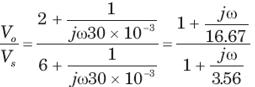Courses

# Frequency Response - MCQ Test

## 20 Questions MCQ Test Mock Tests Electronics & Communication Engineering GATE 2020 | Frequency Response - MCQ Test

Description
This mock test of Frequency Response - MCQ Test for Railways helps you for every Railways entrance exam. This contains 20 Multiple Choice Questions for Railways Frequency Response - MCQ Test (mcq) to study with solutions a complete question bank. The solved questions answers in this Frequency Response - MCQ Test quiz give you a good mix of easy questions and tough questions. Railways students definitely take this Frequency Response - MCQ Test exercise for a better result in the exam. You can find other Frequency Response - MCQ Test extra questions, long questions & short questions for Railways on EduRev as well by searching above.
QUESTION: 1

### A parallel resonant circuit has a resistance of 2 kΩ and half power frequencies of 86 kHz and 90 kHz.1. The value of capacitor is

Solution:

BW = ω2 - ω1 = 2π(90 - 86)k = 8π krad/s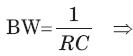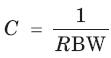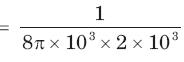= 19.89 nF

QUESTION: 2

### A parallel resonant circuit has a resistance of 2 kΩ and half power frequencies of 86 kHz and 90 kHz.The value of inductor is

Solution: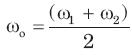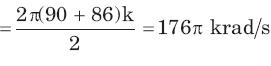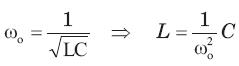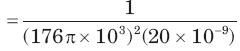= 0.16 mH

QUESTION: 3

### A parallel resonant circuit has a resistance of 2 kΩ and half power frequencies of 86 kHz and 90 kHz.The quality factor is

Solution: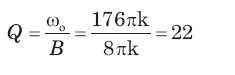QUESTION: 4

A parallel resonant circuit has a midband admittance of 25 x 10-3 S, quality factor of 80 and a resonant frequency of 200 krad/s.The value of R is

Solution:

Ay mid-band frequency Z = R, Y = 1/R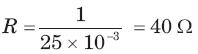QUESTION: 5

A parallel resonant circuit has a midband admittance of 25 x 10-3 S, quality factor of 80 and a resonant frequency of 200 krad/s.The value of C is

Solution: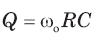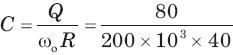= 10 μF

QUESTION: 6

A parallel RLC circuit has R =1 kΩ and C = 1 μF. Thequality factor at resonance is 200. The value of inductor is

Solution: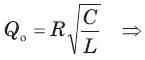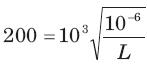⇒ L = 25 μH

QUESTION: 7

A parallel circuit has R =1 k , C = 50 μF and L = 10mH. The quality factor at resonance is

Solution: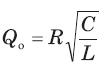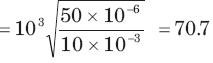QUESTION: 8

A series resonant circuit has an inductor L = 10 mH.The resonant frequency ωo = 106 rad/s and bandwidth is BW = 103 rad/s. The value of R and C will be

Solution: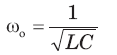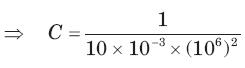= 100pF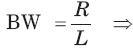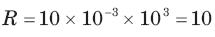QUESTION: 9

A series resonant circuit has L = 1 mH and C = 10μF. The required R for the BW 15 9 . Hz is

Solution: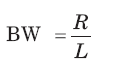⇒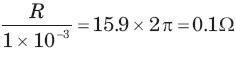QUESTION: 10

For the RLC parallel resonant circuit when R = 8 kΩ , L = 40 mH and C = 0.25 μF, the quality factor Q is

Solution: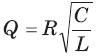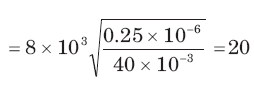QUESTION: 11

The maximum voltage across capacitor would be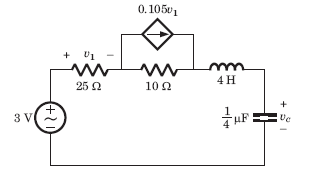Solution:

Thevenin equivalent seen by L - C combination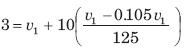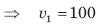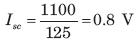Open Circuit : v1 = 0, v∝ = 3V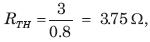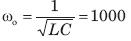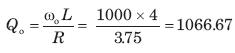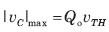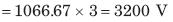QUESTION: 12

For the circuit shown in fig. resonant frequency fo is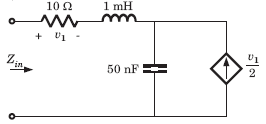Solution:

Applying 1 A at input port V1 = 10 V
voltage across 1 A source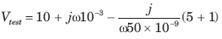Zin = Vtest
At resonance Im {Zin} = 0
⇒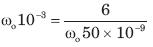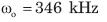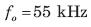QUESTION: 13

For the circuit shown in fig. the resonant frequency fo is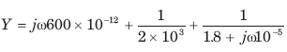Solution: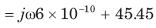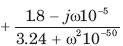At resonance Im {Y} = 0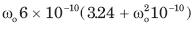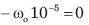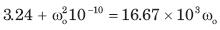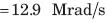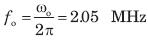QUESTION: 14

The network function of circuit shown in fig. is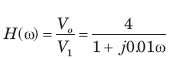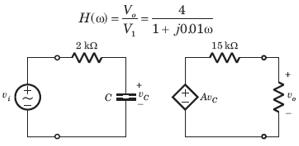Solution: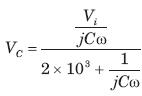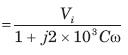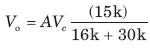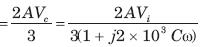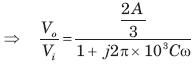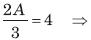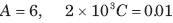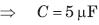QUESTION: 15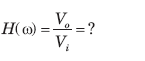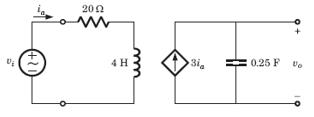Solution: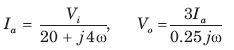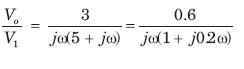QUESTION: 16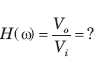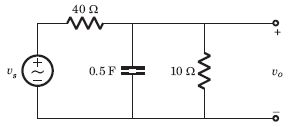Solution: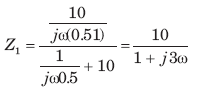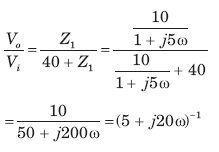QUESTION: 17

The value of input frequency is required to cause again equal to 1.5. The value is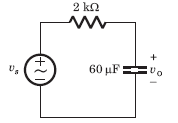Solution: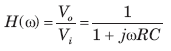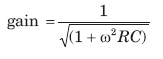For any value of ω, R,C gain ≤ 1.
Thus (D) is correct option.

QUESTION: 18

In the circuit of fig. phase shift equal to -450 is required at frequency ω =20 rad/s . The value of R is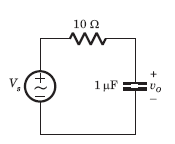Solution: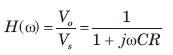phase shift = - tan-1 ωCR = -450°
ωCR = 1,

20 x 1 x 10-6 R = 1 ⇒ R = 50KΩ

QUESTION: 19

For the circuit of fig., Vs is the source voltage and the response is the resistance voltage Vo, R = 30Ω and L = 2H. Suppose the input frequency is adjusted until the gain is equal to 0.6. The value of the frequency is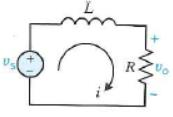Solution: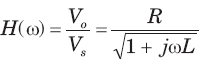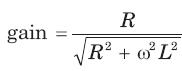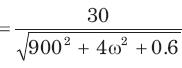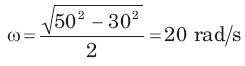QUESTION: 20

Bode diagram of the network function Vo /Vfor the circuit of fig.  is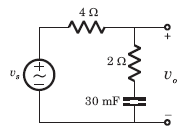Solution: